# Mathematics limits and continuity inter solutions

## Inter maths 1b limits and continuity solutions

Intermediate mathematics 1b chapter 8  limits and continuity solutions for some problems.

Here you can also see the solutions for 1a and 1b some chapters.

You can see the solutions for junior inter maths 1b solutions.

1. Locus

Straight lines sa

Straight lines la

11. Rate measure

You can see the solutions for junior inter maths 1a solutions

3. Matrices

Trigonometric ratios upto transformations 2

### Intermediate maths first year 1b limits and continuity solutions.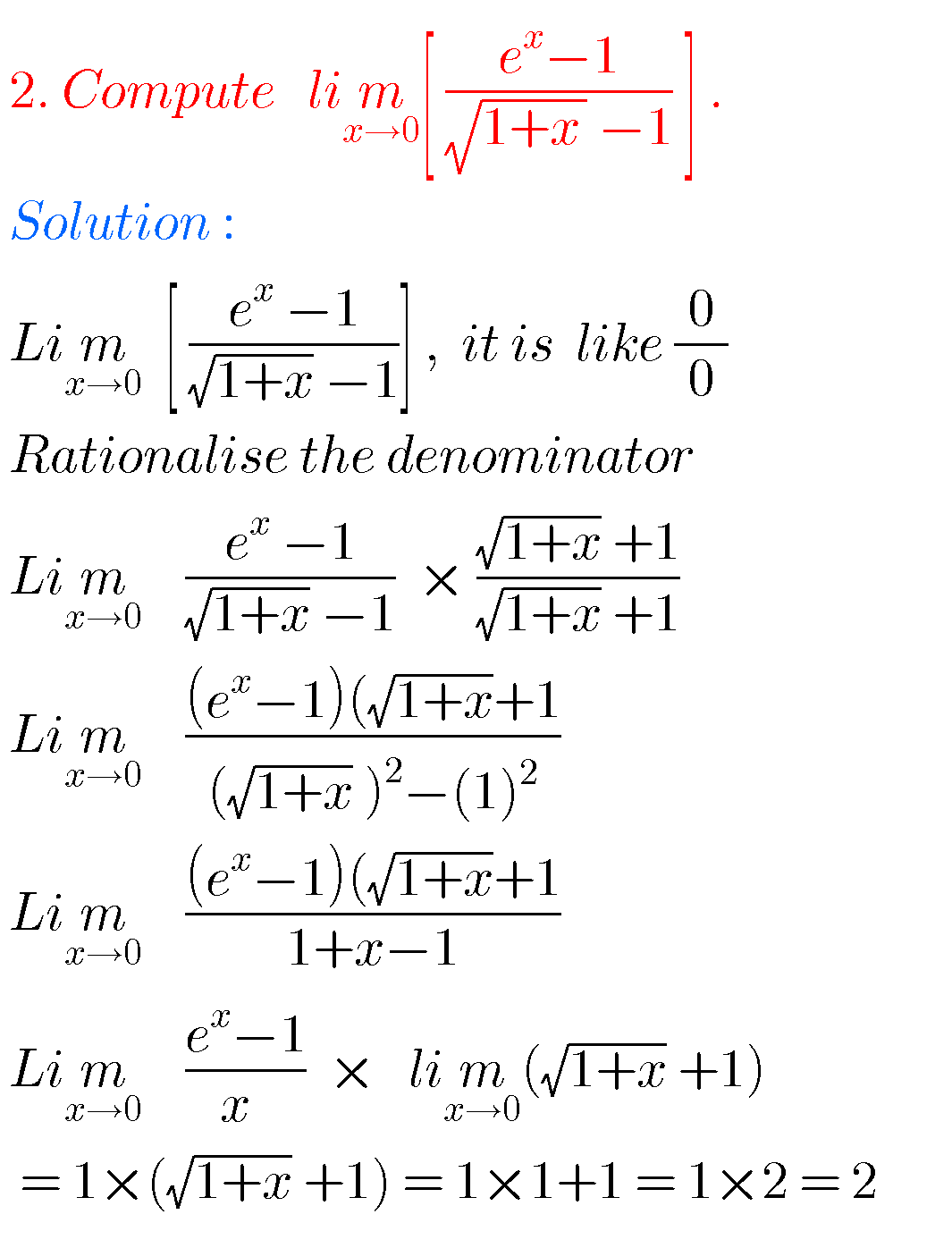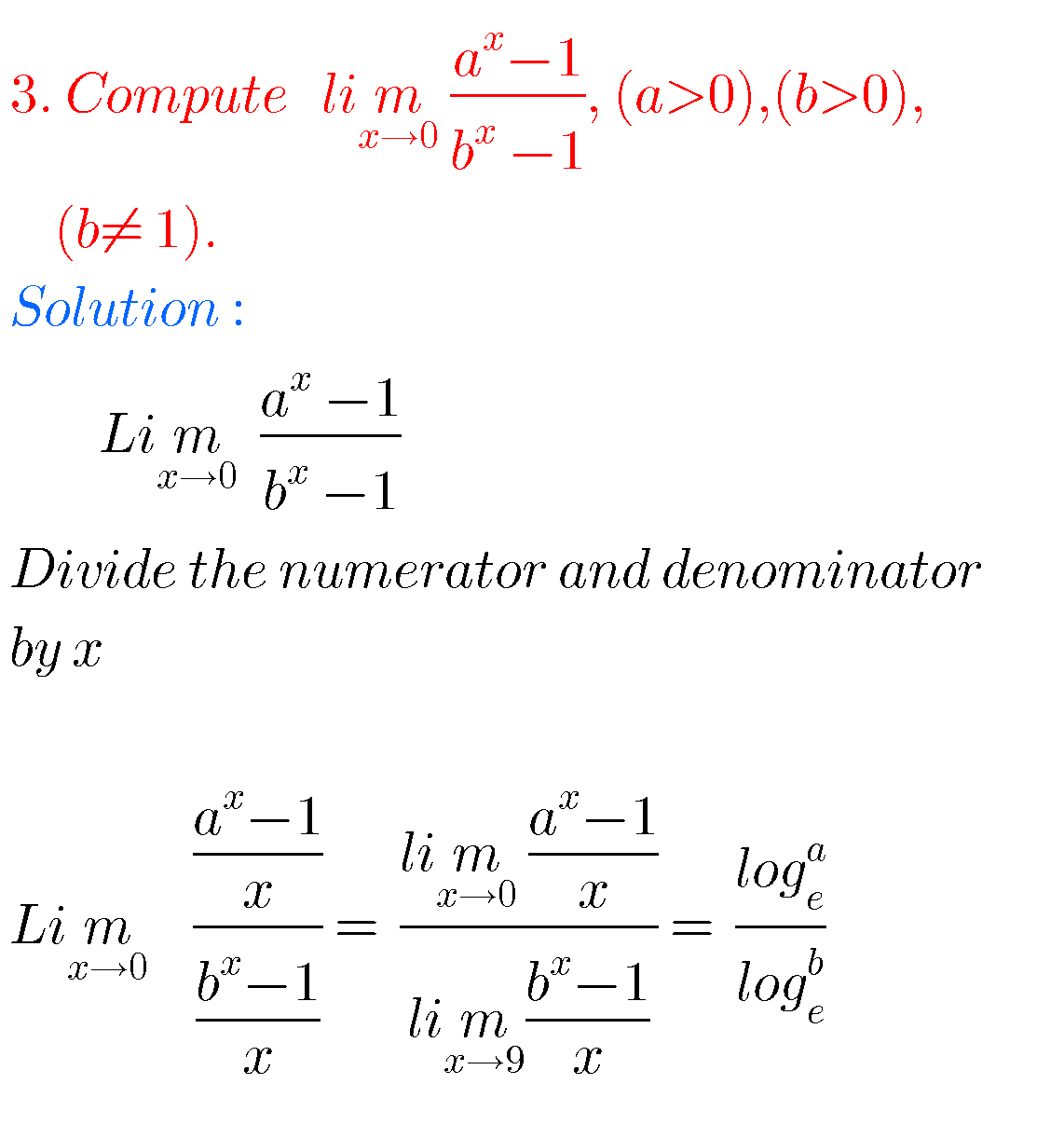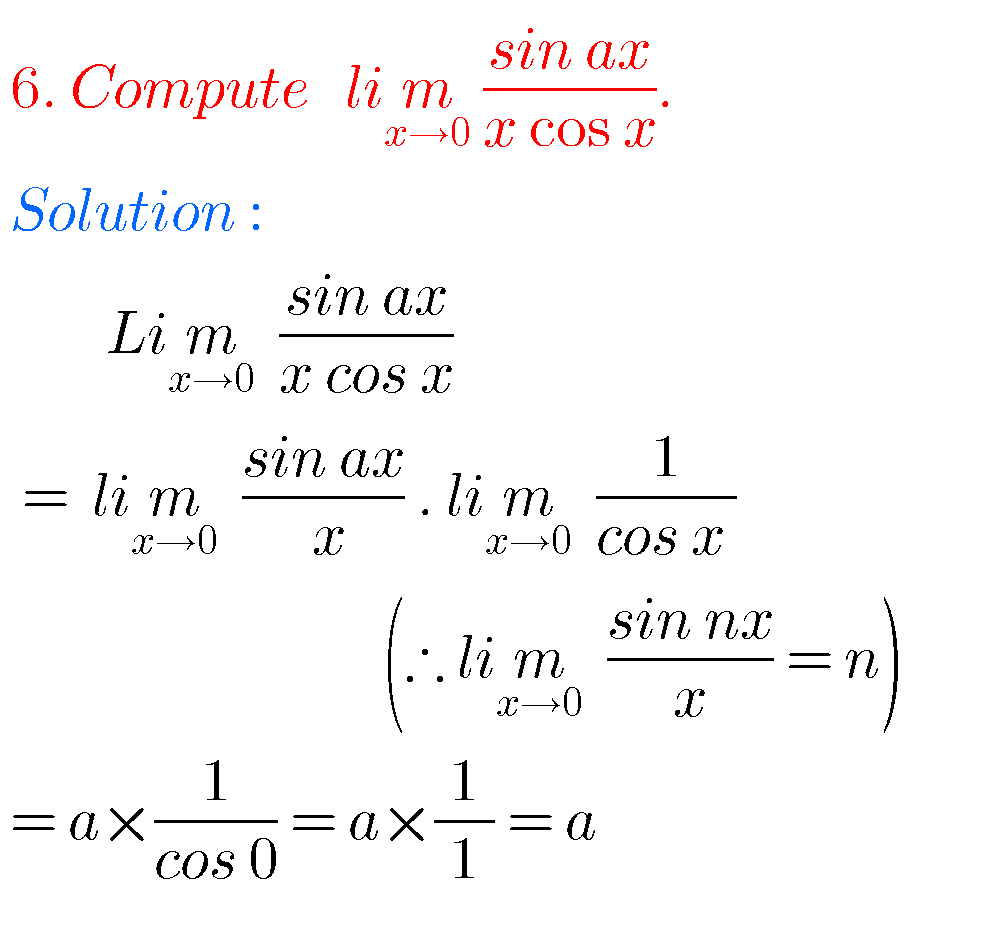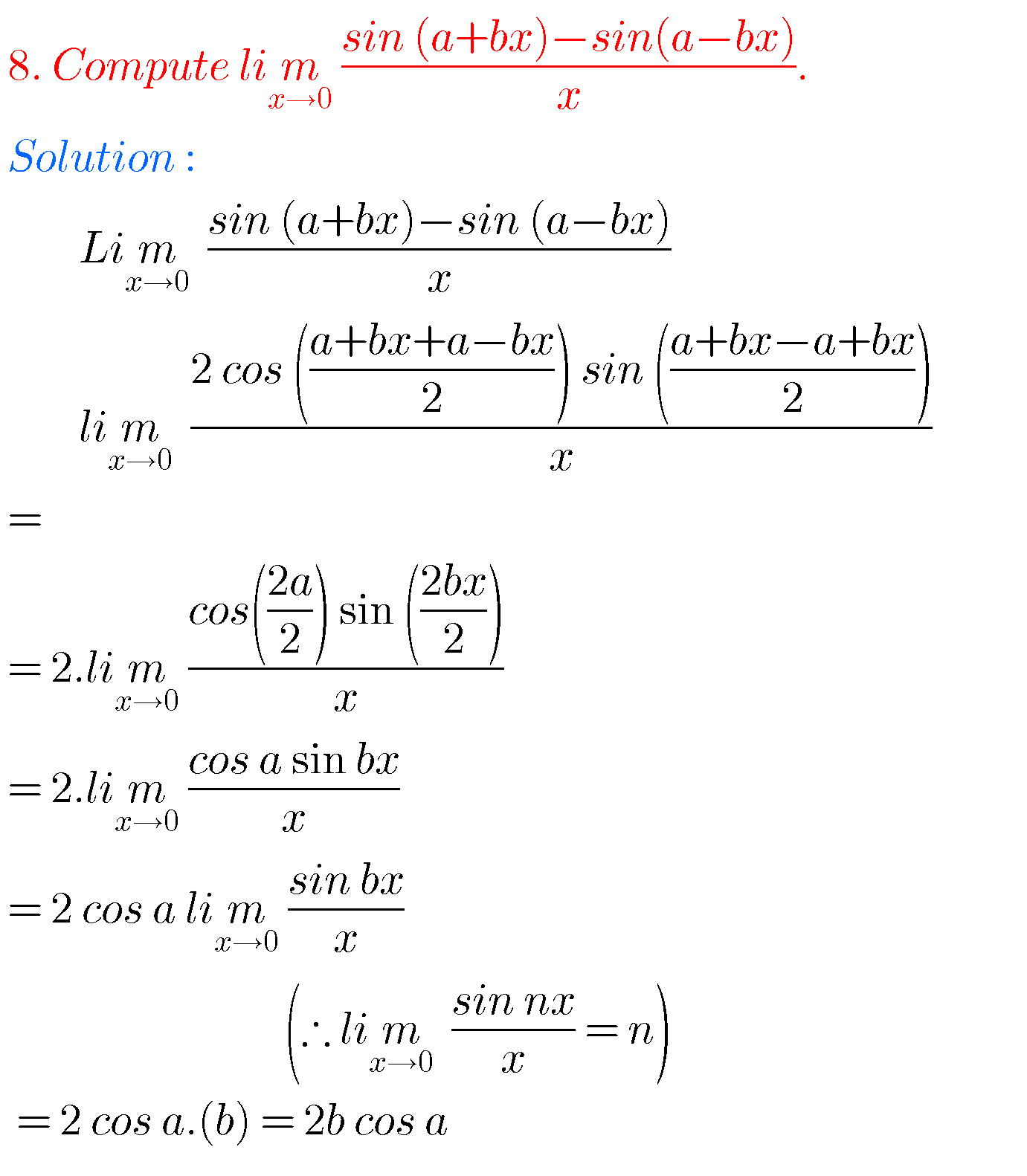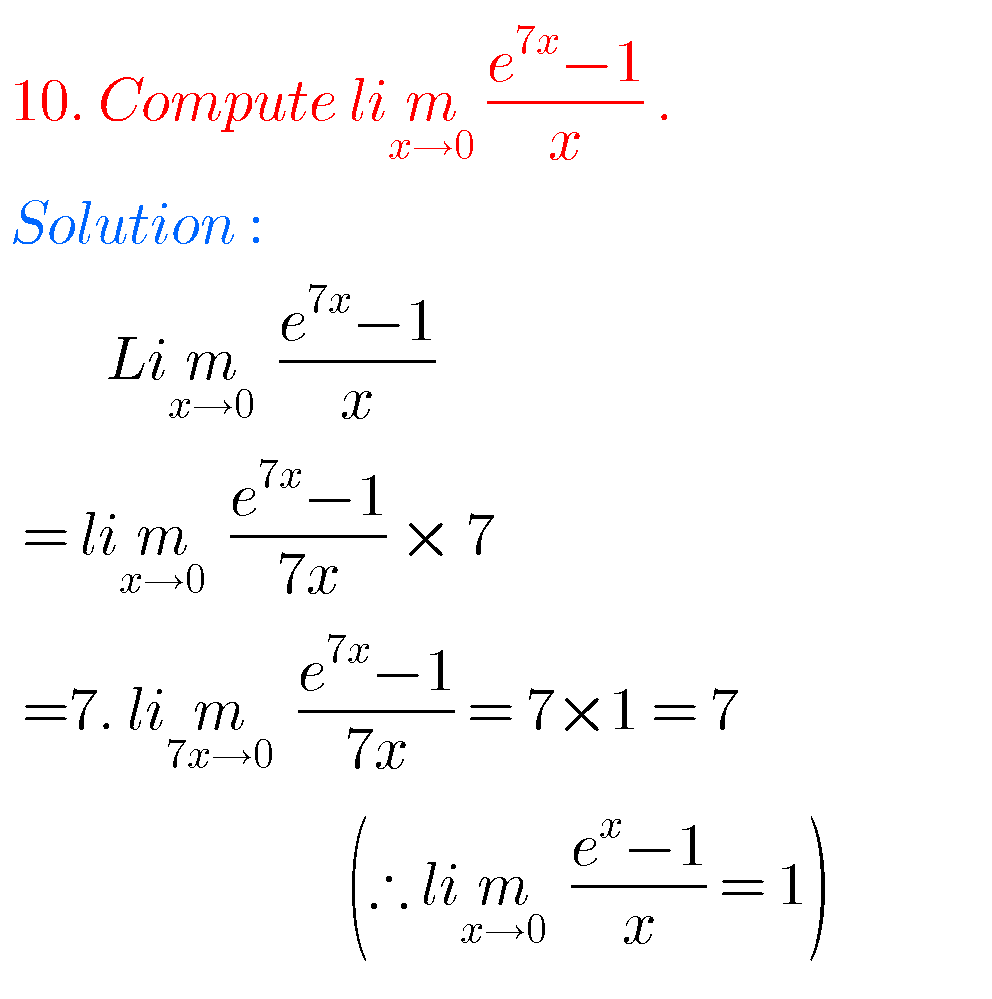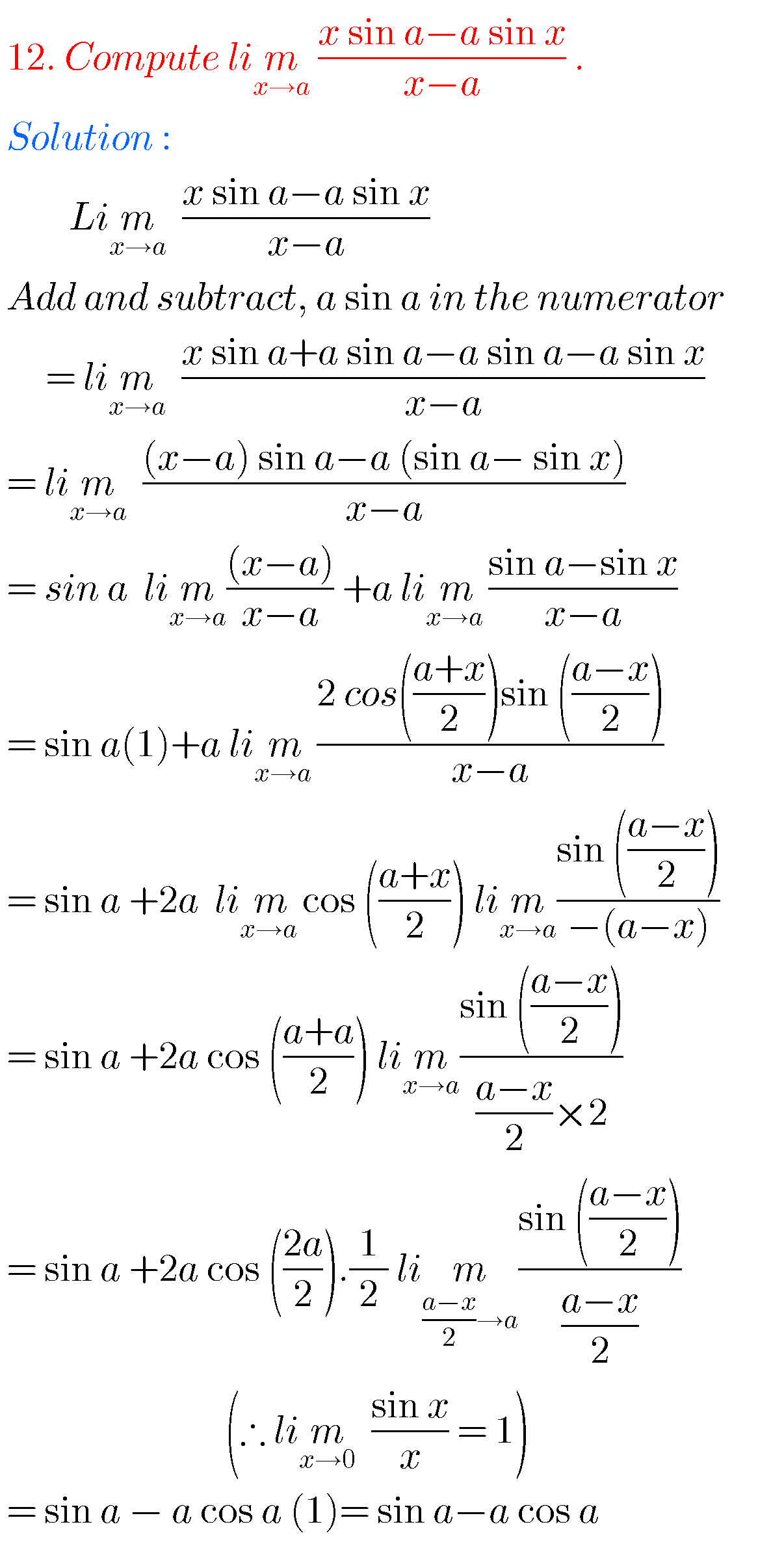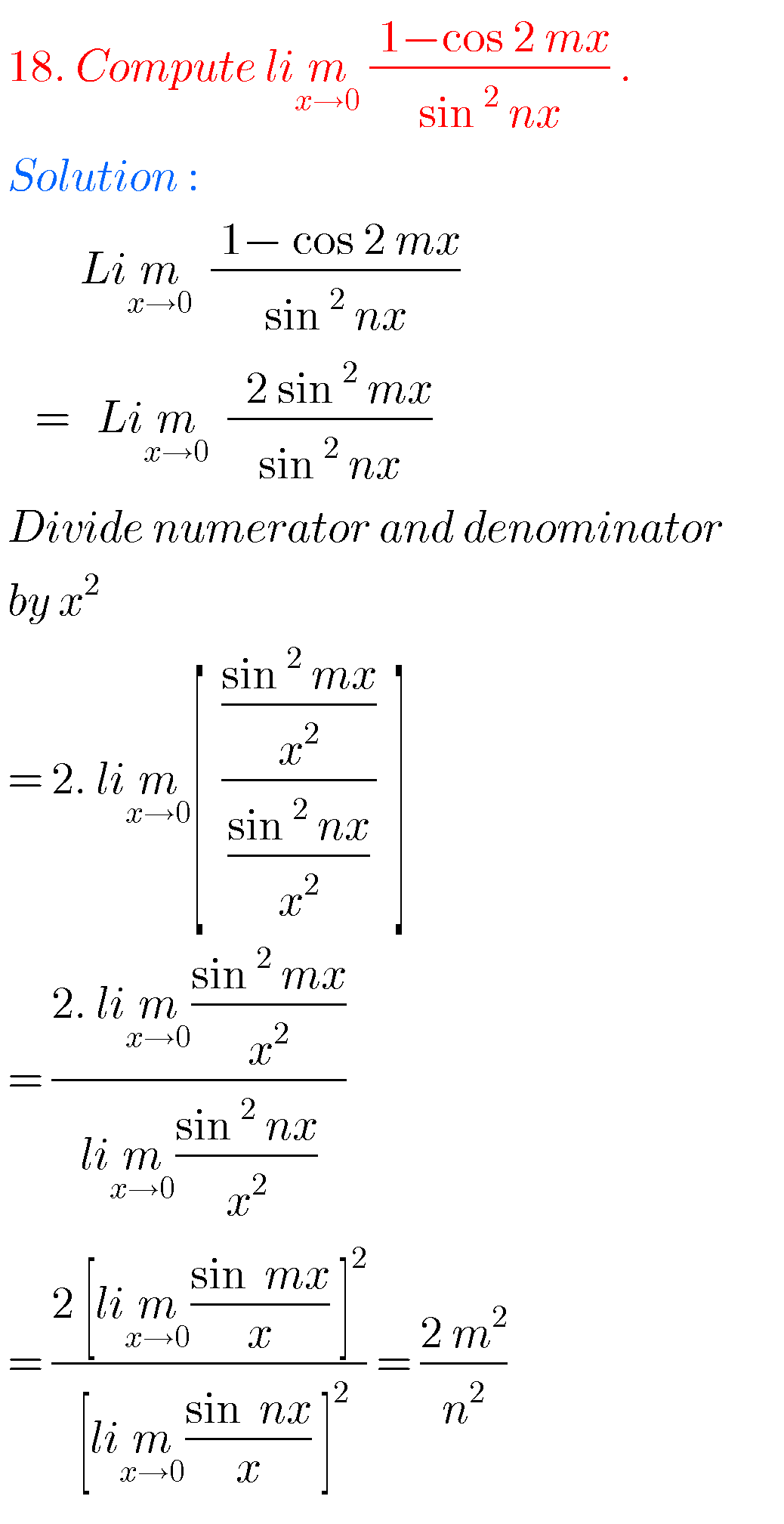##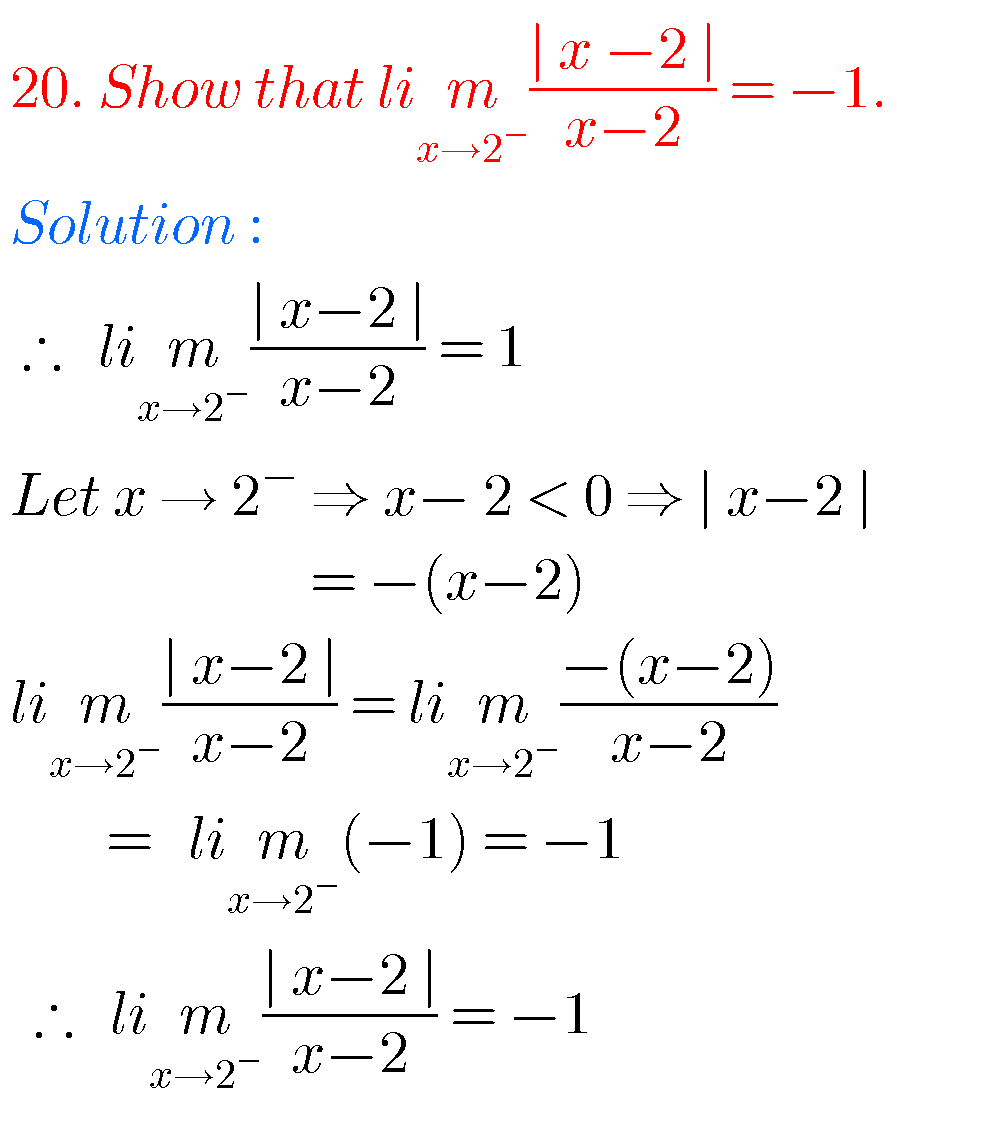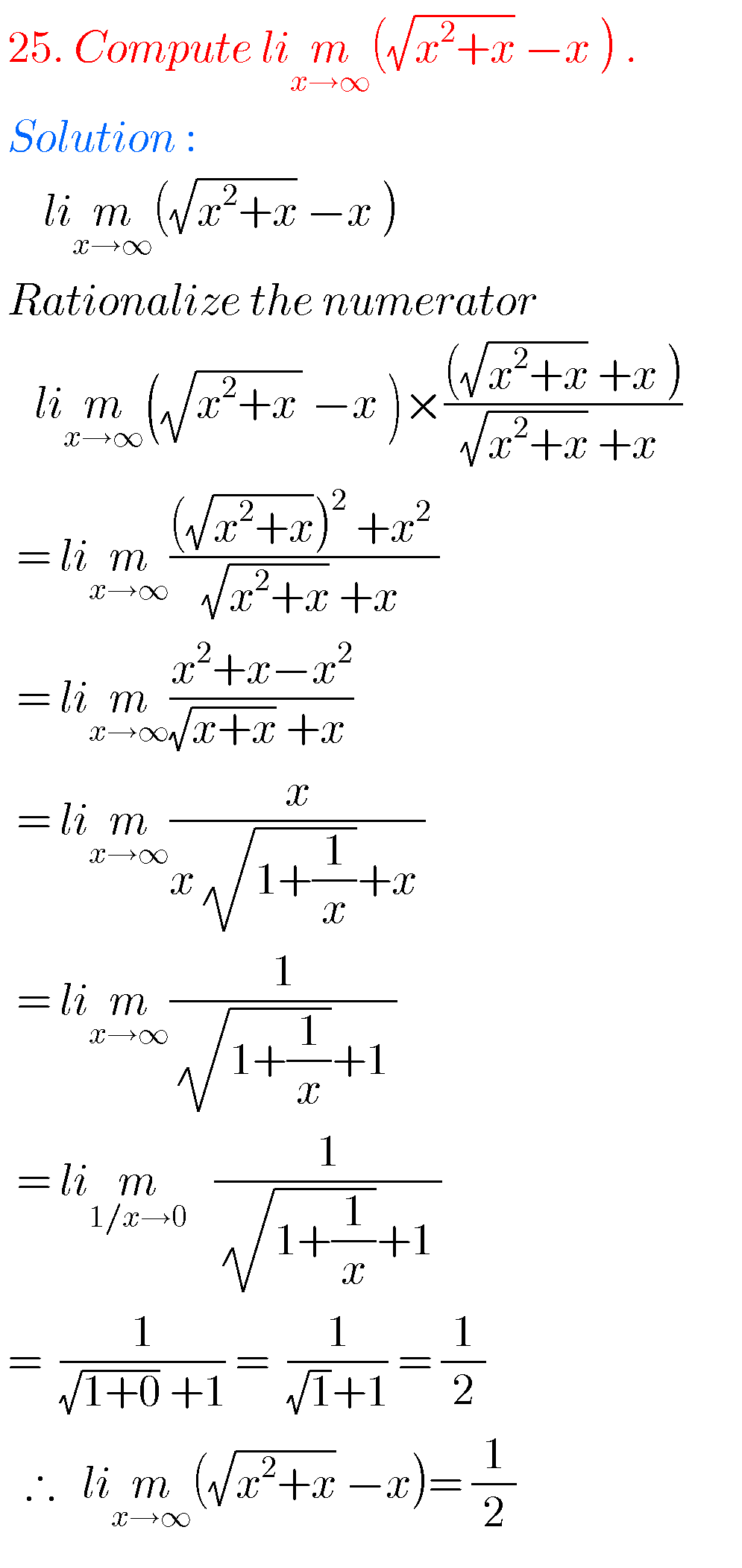## Calculus limits and continuity solutions inter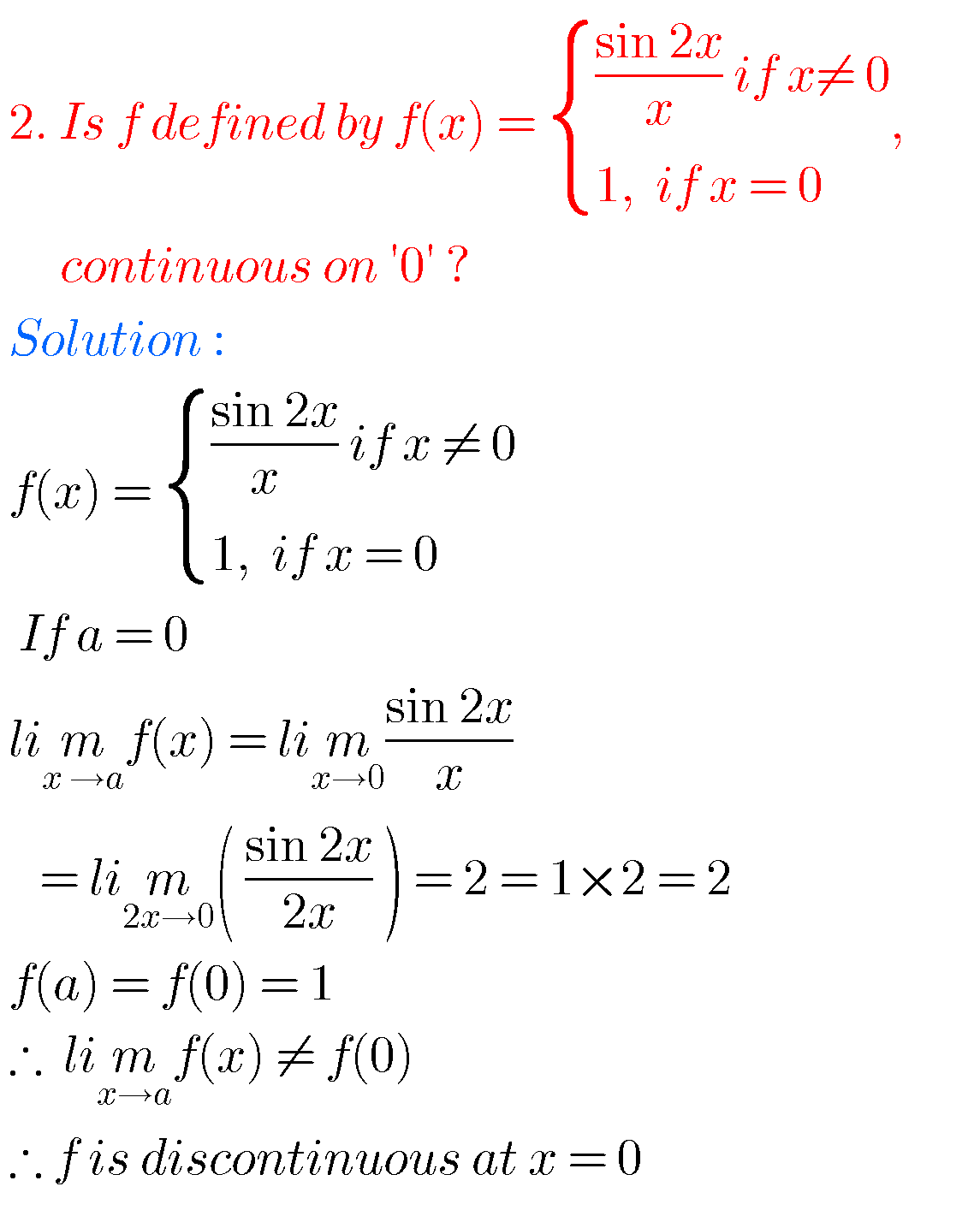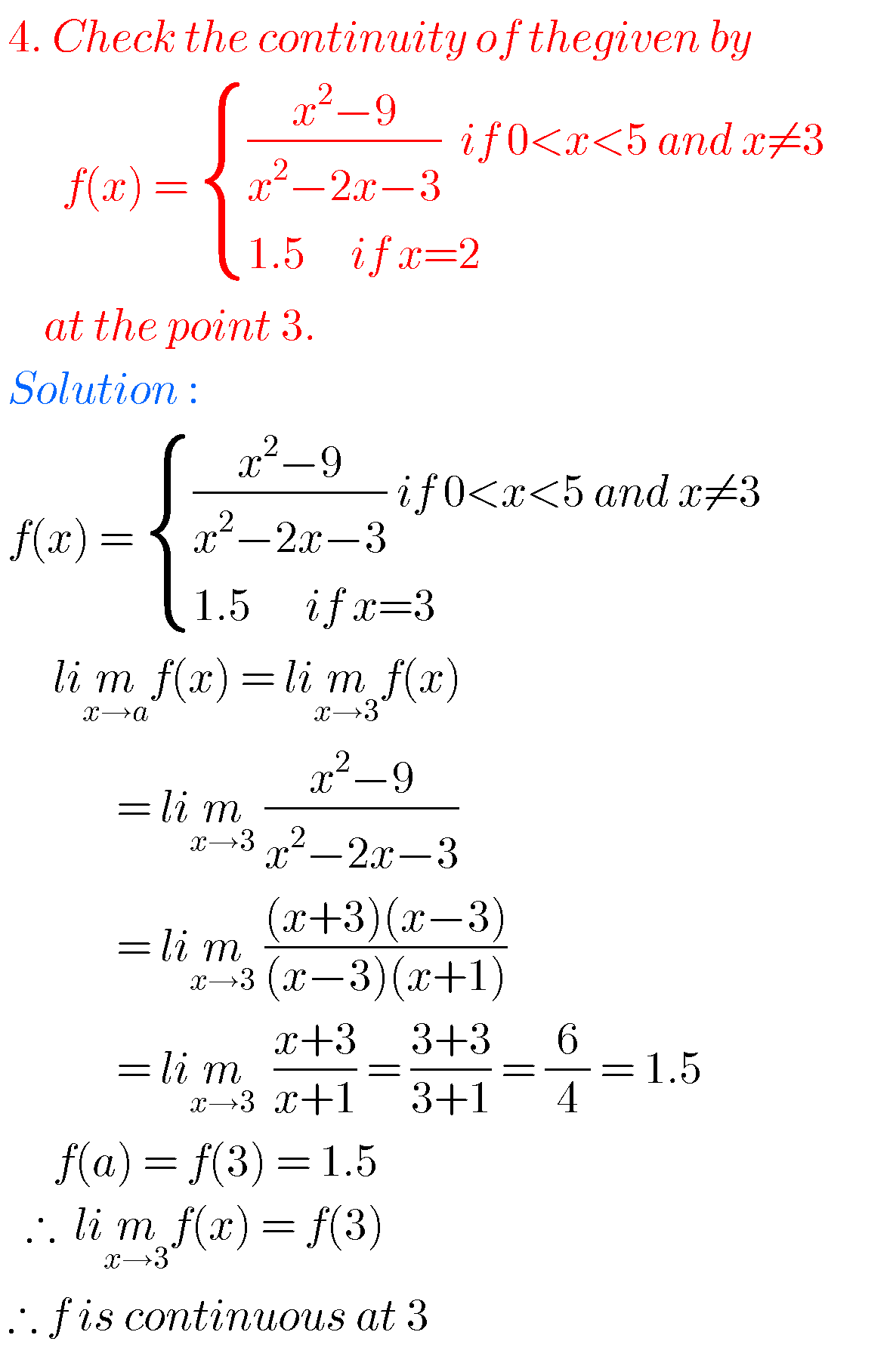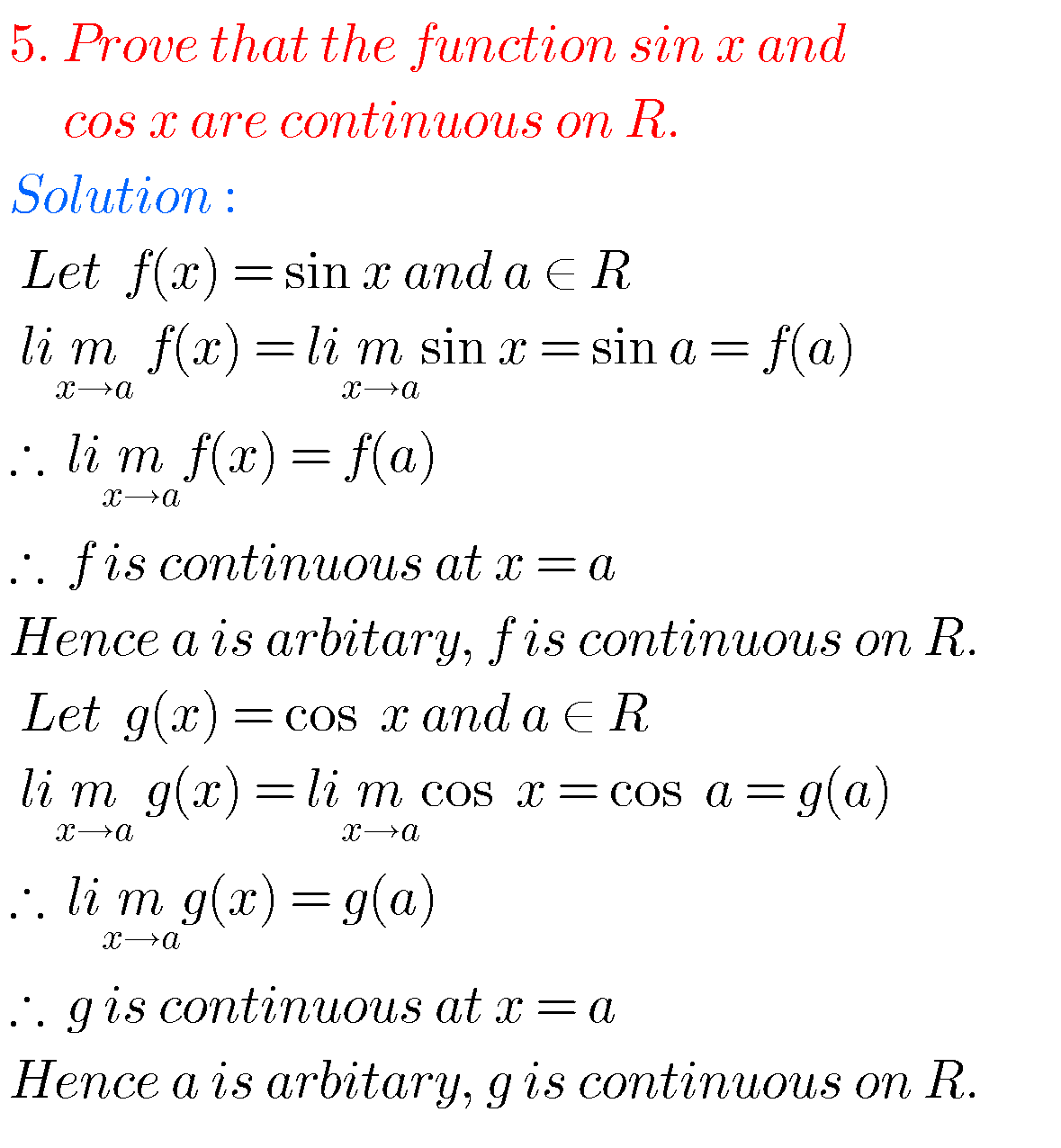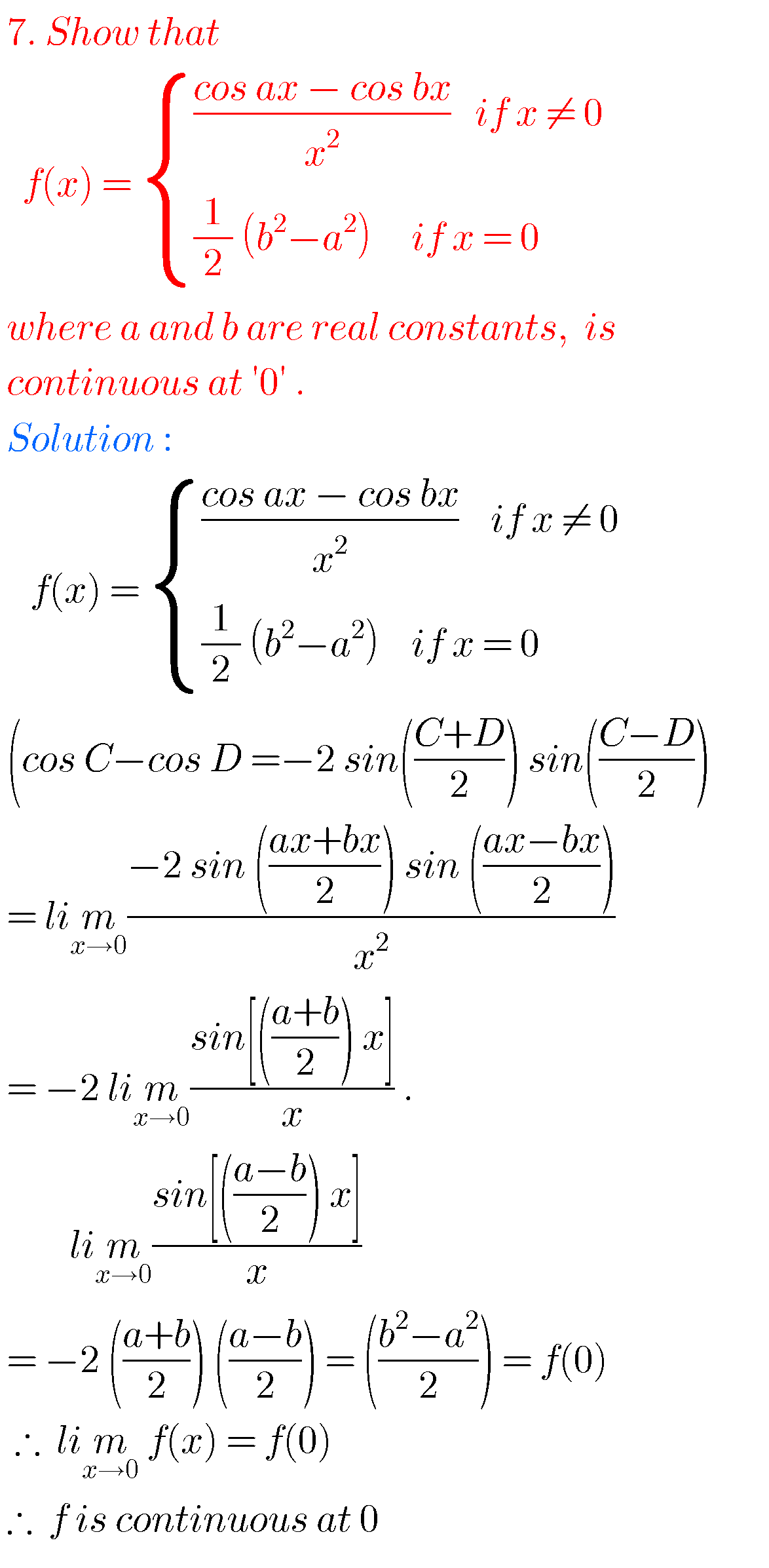Note : Observe the solutions and try them in your own methods.

Maths real numbers solutions class 10